MC A bank needs to store the following information: customer names, customer addresses, city of a branch, number of accounts, account ids and account balances. How many record types do you need to construct a hierarchical database with this information? One incorrect Three correct Four incorrect Five incorrect MC If a hierarchical model contains N:M relationship types that have been integrated by repeating the child nodes where necessary, what are the dangers of updating the database? Slower retrieval of data incorrect Creating data inconsistency correct Creating unnecessary records incorrect All of the above incorrect MC The human resources department of a university wants to make sure that every course has exactly one main professor. How can they implement this constraint using the CODASYL framework? They introduce an extra set type called 'is-main-professor-of' between record types 'professor' and 'course'. correct They introduce an 'is-main-professor' record type between record types 'professor' and 'course' to model the correct relationship. incorrect They introduce 'main professor' as a data item in the record type 'professor'. incorrect It is impossible to model this constraint in CODASYL. incorrect MC In CODASYL, a multivalued composite attribute type can be represented as a: Record type incorrect Data item incorrect Vector incorrect Repeated group correct MC How can the CODASYL framework correctly model a family tree, taking into account that every child has to have (at least) two parents? Use a set type 'is parent of' between record types 'parent' and 'child'. incorrect Use a dummy record type 'is parent of' between record types 'parent' and 'child'. correct List the parents as a vector in the data item set of every child. incorrect We cannot model this constraint with the CODASYL framework. incorrect MCGiven this ER model between 'Bungalow' and 'Reservation', how many of the 4 cardinalities can be enforced in the CODASYL model? 1 incorrect 2 incorrect 3 correct 4 incorrect MC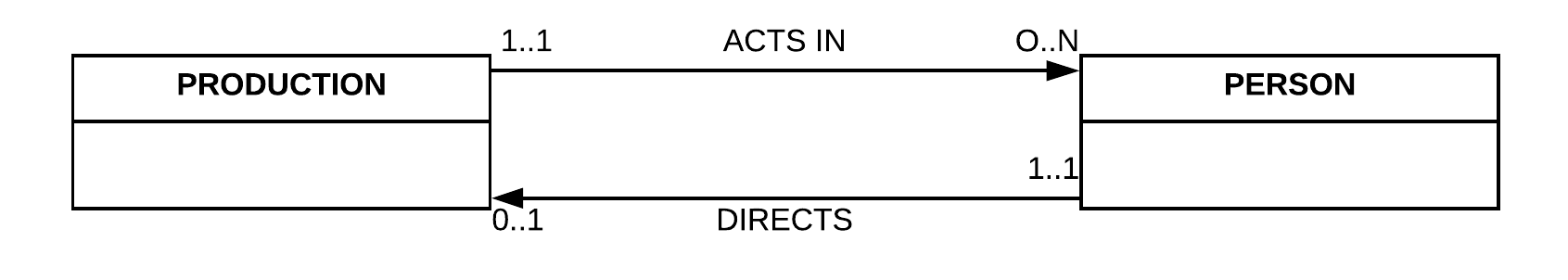How many of these above cardinalitites can be enforced in the CODASYL model? 5 incorrect 6 incorrect 7 correct 8 incorrect MC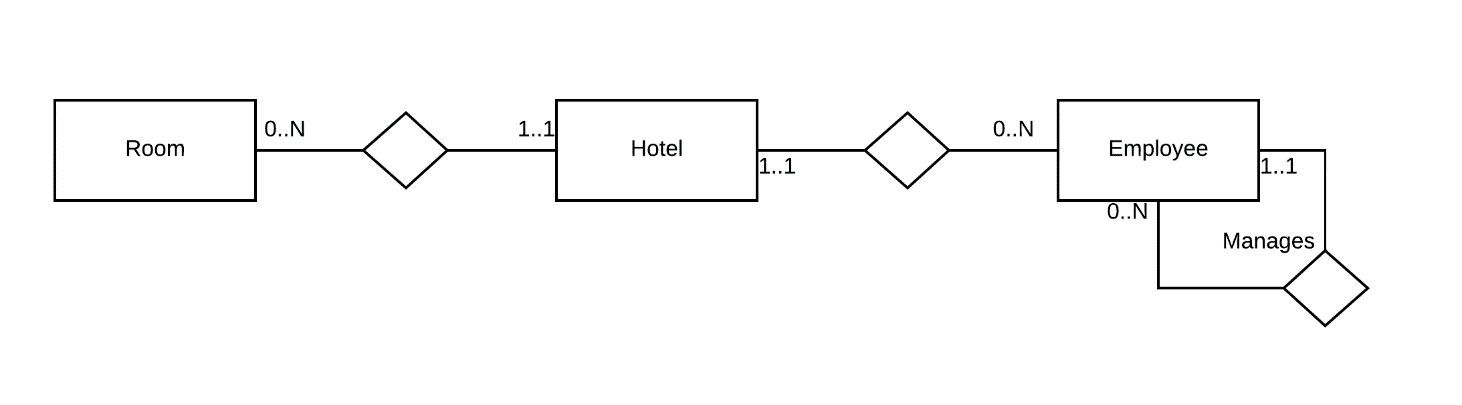If we would model the above EER model as a hierarchical model, how many record types would we need? 3 incorrect 4 correct 5 incorrect 6 incorrect MC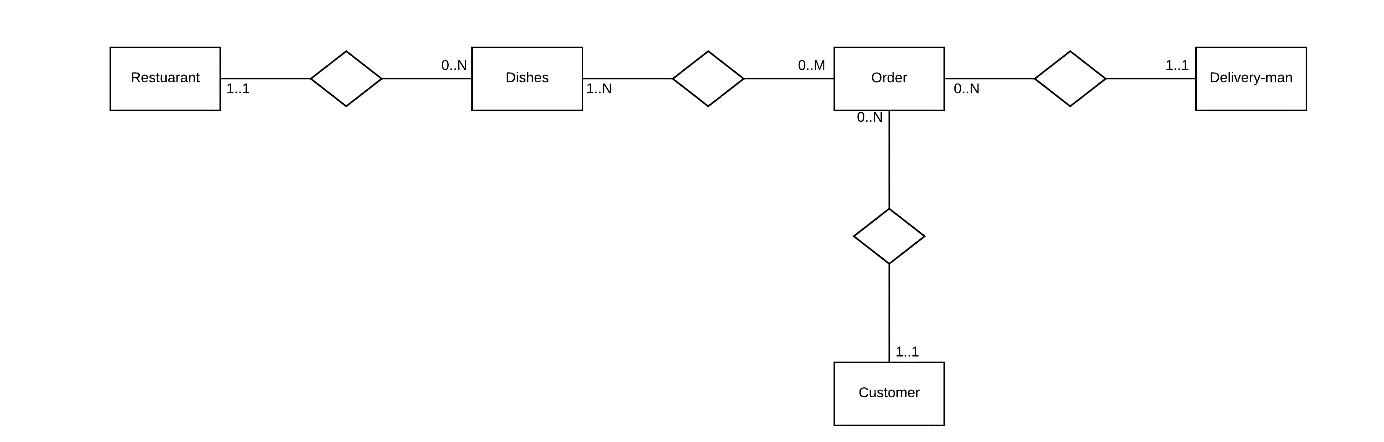If we would turn the above EER model into a hierarchical model, how many record types would we have to introduce? 5 incorrect 6 correct 7 incorrect 8 incorrect MC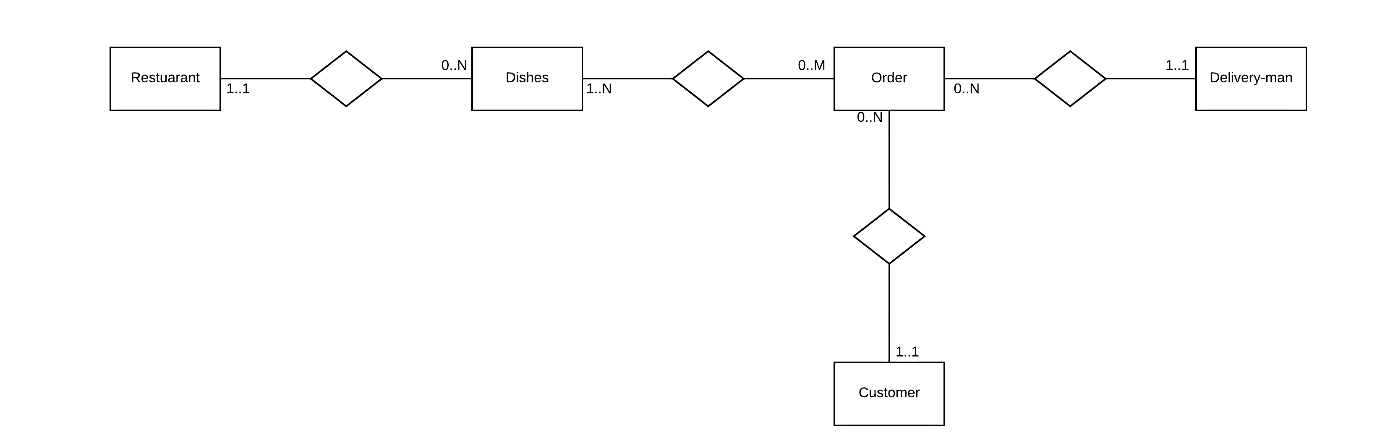Which of the following statements on turning the above EER model into a hierarchical model is correct ? All cardinalities can be enforced. incorrect We have to introduce a virtual child record type to model the relationship between 'Dishes' and 'Order'. correct We have to introduce a virtual child record type to model the relationship between 'Restaurant' and 'Dishes'. incorrect We cannot enforce that an order has exactly one customer. incorrect MC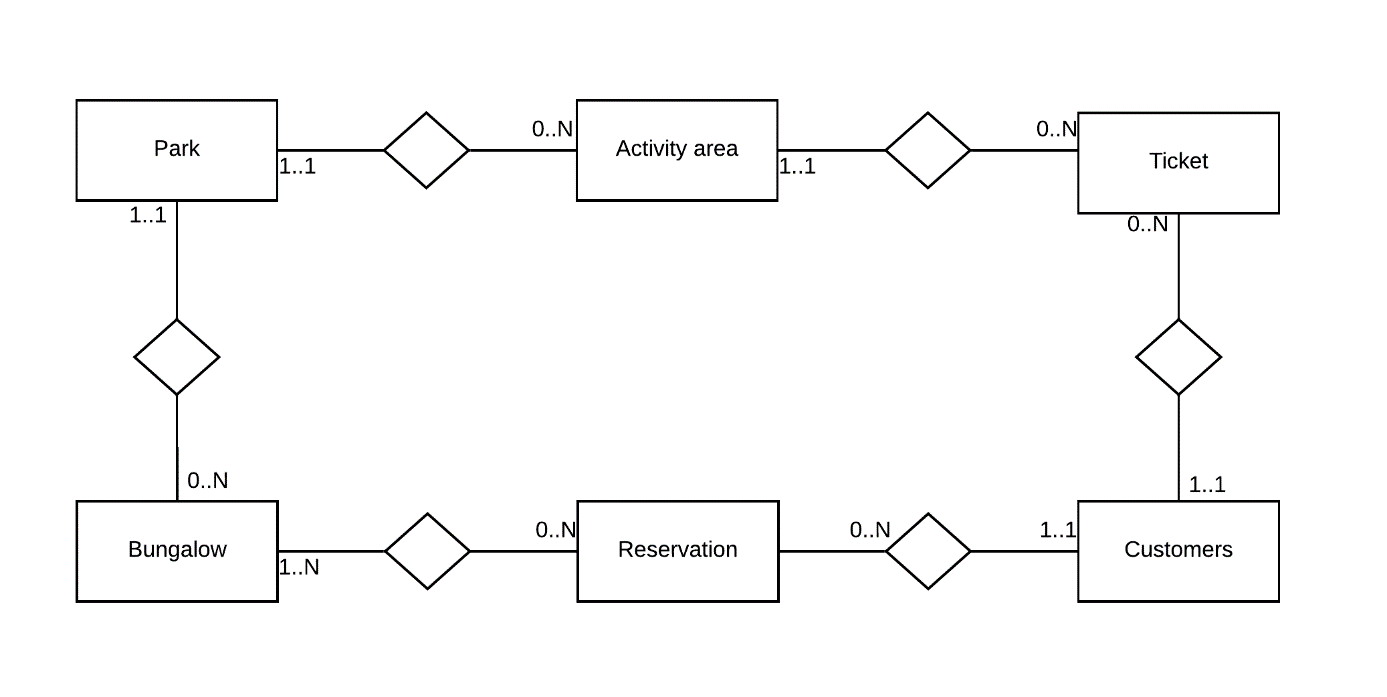Which of the following statements on turning the above EER model into a CODASYL model is correct? We have to introduce 6 record types. incorrect We cannot enforce that a reservation needs at least one customer. correct We have to introduce 8 record types. incorrect All of the above statements are correct. incorrect MC How can the CODASYL framework model a person's e-mail address (assuming a person can have more than one e-mail address)? Introduce a virtual child for each mail address. incorrect Use a repeated group. incorrect Use a vector. correct We cannot model this in the CODASYL framework. incorrect MC Which statement is CORRECT? The hierarchal model allows you to introduce multiple relationship types between the same set of record types. incorrect In both a CODASYL and hierarchical model, the child can be disconnected from its parent. incorrect A CODASYL model is usually represented in a network or Bachman diagram. correct In the CODASYL framework, a relationship type is used to connect the record types. incorrect MC Which statement is NOT CORRECT? Legacy databases are behind us and irrelevant nowadays. correct A root record type sits at the top of the hierarchy. incorrect A leaf record type sits at the bottom of the hierarchy. incorrect A hierarchical DBMS has a very inefficient DML. incorrect